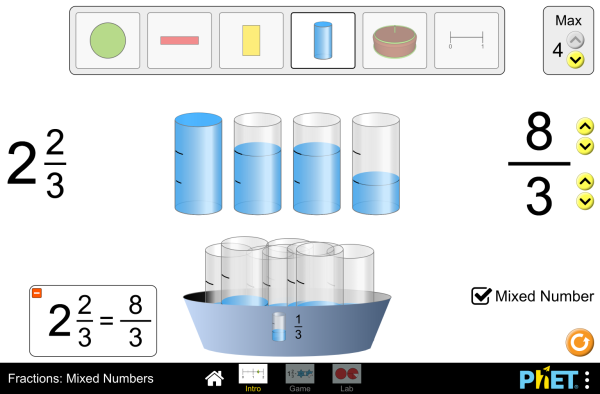# 分数：带分数下载嵌入代码 关闭 嵌入模拟程序控制 你可以通过改变嵌入的代码改变程序的宽度和高度 插入一张图片，当点击这个图片时启动仿真程序。

• 分数
• 等值分数
• 带分数
• 数轴

### 示范 学习目标

• Predict and explain how changing the numerator of a fraction affects the fraction's value
• Predict and explain how changing the denominator of a fraction affects the fraction's value
• Convert between a picture of a fraction, an improper fraction, and a mixed number
• Build matching fractions using numbers and pictures

### 标准对齐

#### Common Core - Math

3.NF.A.1
Understand a fraction 1/b as the quantity formed by 1 part when a whole is partitioned into b equal parts; understand a fraction a/b as the quantity formed by a parts of size 1/b.
3.NF.A.2
Understand a fraction as a number on the number line; represent fractions on a number line diagram.
3.NF.A.2b
Represent a fraction a/b on a number line diagram by marking off a lengths 1/b from 0. Recognize that the resulting interval has size a/b and that its endpoint locates the number a/b on the number line.
5.NF.B.3
Interpret a fraction as division of the numerator by the denominator (a/b = a ÷ b). Solve word problems involving division of whole numbers leading to answers in the form of fractions or mixed numbers, e.g., by using visual fraction models or equations to represent the problem. For example, interpret 3/4 as the result of dividing 3 by 4, noting that 3/4 multiplied by 4 equals 3, and that when 3 wholes are shared equally among 4 people each person has a share of size 3/4. If 9 people want to share a 50-pound sack of rice equally by weight, how many pounds of rice should each person get? Between what two whole numbers does your answer lie?

### 教师指南仿真程序控制概述,简化模型,学生视角的思考 ( PDF ).

### 教师提交的活动

Games Remote Lesson ideasTrish Loeblein K-5

Remote

Building Fraction Sense Using “Fractions Intro PhET Simulation”Philippe Guegan K-5

SECUNDARIA: Alineación PhET con programas de la SEP México (2011 y 2017)Diana López 高中

PRIMARIA: Alineación con programas de la SEP México (2011 y 2017)Diana López K-5

Mixed Number Intro Sharifa Footman K-5 家庭作业

### HTML5 程序：

iOS 8+ Safari

Android:

Chromebook:

Windows 系统:

Macintosh 系统:
OS 10.9.5 或之后的系统版本，Safari 9+版本，或者火狐浏览器，谷歌浏览器的最新版本均支持我们的程序。

Linux 系统:

### Java and Flash 程序：

Java 与 Flash程序可以在PC端，mac端，及linux系统上运行。具体的系统配置要求如下：

Windows 系统:
Windows 10, 8.1, 7

Macintosh 系统:
Mac OS X 10.9.5+

64位浏览器（例如Safari，火狐，谷歌等）

Linux 系统:
Oracle Linux 5.5+1
Oracle Linux 6.x (32位), 6.x (64位)2
Oracle Linux 7.x (64位)2
Red Hat Enterprise Linux 5.5+1, 6.x (32位), 6.x (64位)2
Ubuntu Linux 12.04 LTS, 13.x
Suse Linux Enterprise Server 10 SP2+, 11.x

• Sam Reid (original developer)
• Martin Veillette (developer)
• Michael Dubson
• Trish Loeblein
• Ariel Paul
• Kathy Perkins
• Vincent Davis
• Michael Moorer
• Dusty Cole
• almond-0.2.9.js
• base64-js-1.2.0.js
• FileSaver-b8054a2.js
• font-awesome-4.5.0
• game-up-camera-1.0.0.js
• he-1.1.1.js
• himalaya-0.2.7.js
• jama-1.0.2
• jquery-2.1.0.js
• lodash-4.17.4.js
• pegjs-0.7.0.js
• seedrandom-2.4.2.js
• text-2.0.12.js
• TextEncoderLite-3c9f6f0.js